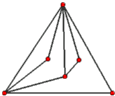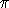# Pseudo-Triangulations Project Page by Lutz Kettner

## Table ofContents## Introduction

A pseudo-triangle is a planar polygon that has exactly three convex vertices, called corners, with internal angles less than. A pseudo-triangulation for a set P of n points in the plane partitions the convex hull of P into pseudo-triangles whose vertex set is exactly P, see the Figure above for a small example. A minimum pseudo-triangulation uses the smallest number of pseudo-triangles, which is always n-2.

## Degree Bounds for Pseudo-Triangulations

Every point set in general position has a minimum pseudo-triangulation whose maximum vertex degree is five, see [Kettner et al. 01] for the constructive proof. This bound is tight, since every pseudo-triangulation for the corners of a regular 11-gon and its center as twelfth point needs a degree-five vertex.

Furthermore, every point set in general position also has a minimum pseudo-triangulation whose maximum face degree is four (i.e. each face of this pseudo-triangulation has at most four vertices).

## Enumerating Pseudo-Triangulations

Oswin Aichholzer et al. built a data base of order types for small point sets for up to ten points. In [Brönnimann et al. 01] we describe an algorithm to enumerate all minimum pseudo-triangulations for a point set. We used two independent implementations, one by myself can be found below and one by Hervé Brönnimann can be found here, to count the number of minimum pseudo-triangulations for all order types for up to ten points based on above data base.

The counting results are summarized in the following table. For the point set(s) with the maximal number of minimum pseudo-triangulations we give the number in the data base (table column order type # for max starting with 0 for the first set, which is in line with my tools provided below, but in contrast with Oswin Aichholzer's counting that starts with 1). The numbers are linked to the point coordinates. An example picture is available too. The last column gives access to the full binary data following the format of the order type data base.

We further observe in the data sets that the number of minimum pseudo-triangulations is always greater than the number of triangulations except if the points are in convex position where the numbers are identical. Furthermore, the points in convex position minimize the number of minimum pseudo-triangulations, which has actually now been proven to be correct for all number of points by O. Aichholzer, F. Aurenhammer, H. Krasser, and B. Speckmann in CCCG 2002, see here.

 #points #order types min #pseudo-tr. max #pseudo-tr. order type # for max data files 3 1 1 1 set 0 (picture) pseudo03.b08 4 2 2 3 set 1 (picture) pseudo04.b08 5 3 5 13 set 2 (picture) pseudo05.b08 6 16 14 76 set 14 (picture) pseudo06.b08 7 135 42 485 set 124 (picture) pseudo07.b16 8 3315 132 3555 set 2990 (picture) set 3198 (picture) pseudo08.b16 9 158817 429 27874 set 151720 (picture) pseudo09.b16 10 14309547 1430 234160 set 13413893 (picture) set 13812359 (picture) pseudo10.b32.gz

## Software

• Pseudo-Triangulation Workbench (ptw)

The Pseudo-Triangulation Workbench (ptw) is a research tool to investigate pseudo-triangulations of points in the plane. It allows to create point sets, compute pseudo-triangulations using various algorithms, interact with them using flips, highlight interesting properties using different color schemes, and enumerate all pseudo-triangulations for a given point set. The enumeration can also be used with a branch&bound version to test hypothesis, such as minimal vertex degree. Currently, the algorithms and enumeration for pseudo-triangulations create only minimal pseudo-triangulations. Some support exists for normal triangulations, such as creation and diagonal flips. Command-line tools allow the enumeration of points sets, for example taken from the data base of order types for small point sets, without graphical user interface.

## Links

Do you have a link you want to see here? Please write me.

Lutz Kettner () 12.09.2001